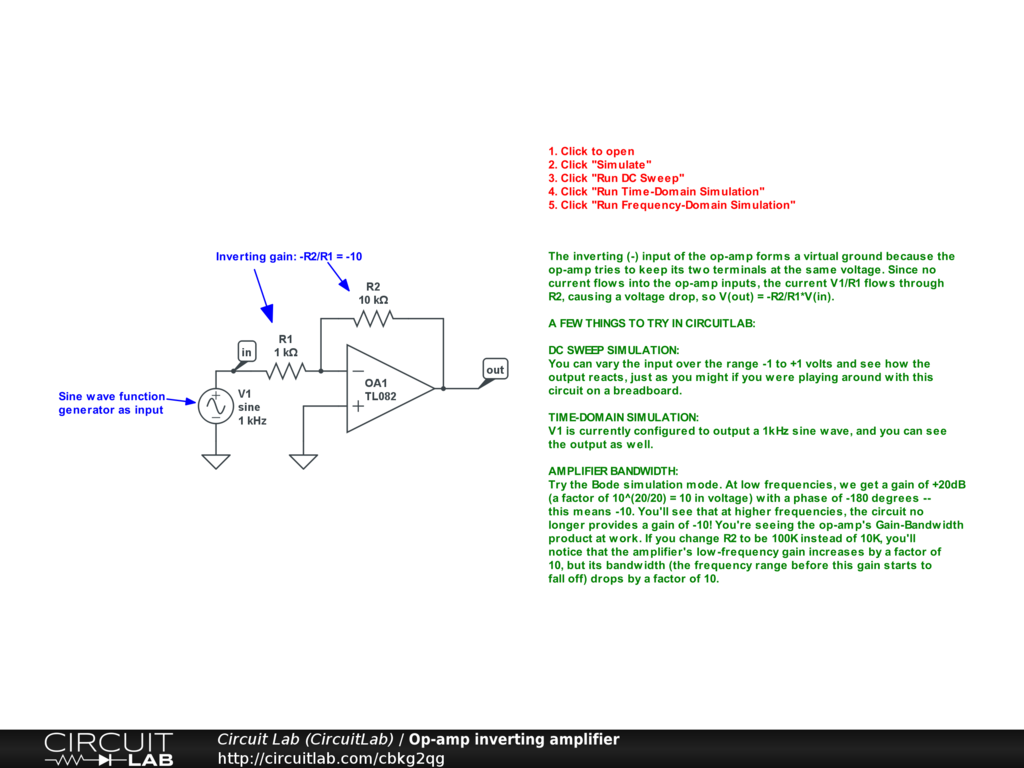Created by Created November 13, 2011 Last modified June 08, 2017 Tags

### Summary

Two resistors plus an op-amp form a gain-of-10 amplifier.

### Description

The op-amp tries to keep its two input terminals at the same voltage, so as the non-inverting (+) input changes, the op-amp tries to output whatever voltage it can to get the inverting (-) input to the same voltage. Since the voltage at the inverting input is set to 1/10th of V(out) by the resistor voltage divider ratio R1/(R2+R1), V(out)=R1/(R2+R1)*V(in).

#### A few things to try in CircuitLab

##### DC sweep simulation

You can vary the input over the range -1 to +1 volts and see how the output reacts, just as you might if you were playing around with this circuit on a breadboard.

##### Time simulation

V1 is currently configured to output a 1kHz sine wave, and you can see the output as well.

##### Amplifier bandwidth

Try the Bode simulation mode. At low frequencies, we get a gain of +20dB (a factor of 10^(20/20) = 10 in voltage) with a phase of 0 degrees. You'll see that at higher frequencies, the circuit no longer provides a gain of 10! You're seeing the op-amp's Gain-Bandwidth product at work. If you change R2 to be 99K instead of 9K, you'll notice that the amplifier's low-frequency gain increases by a factor of 10, but its bandwidth (the frequency range before this gain starts to fall off) drops by a factor of 10.

Op-amp inverting amplifier: